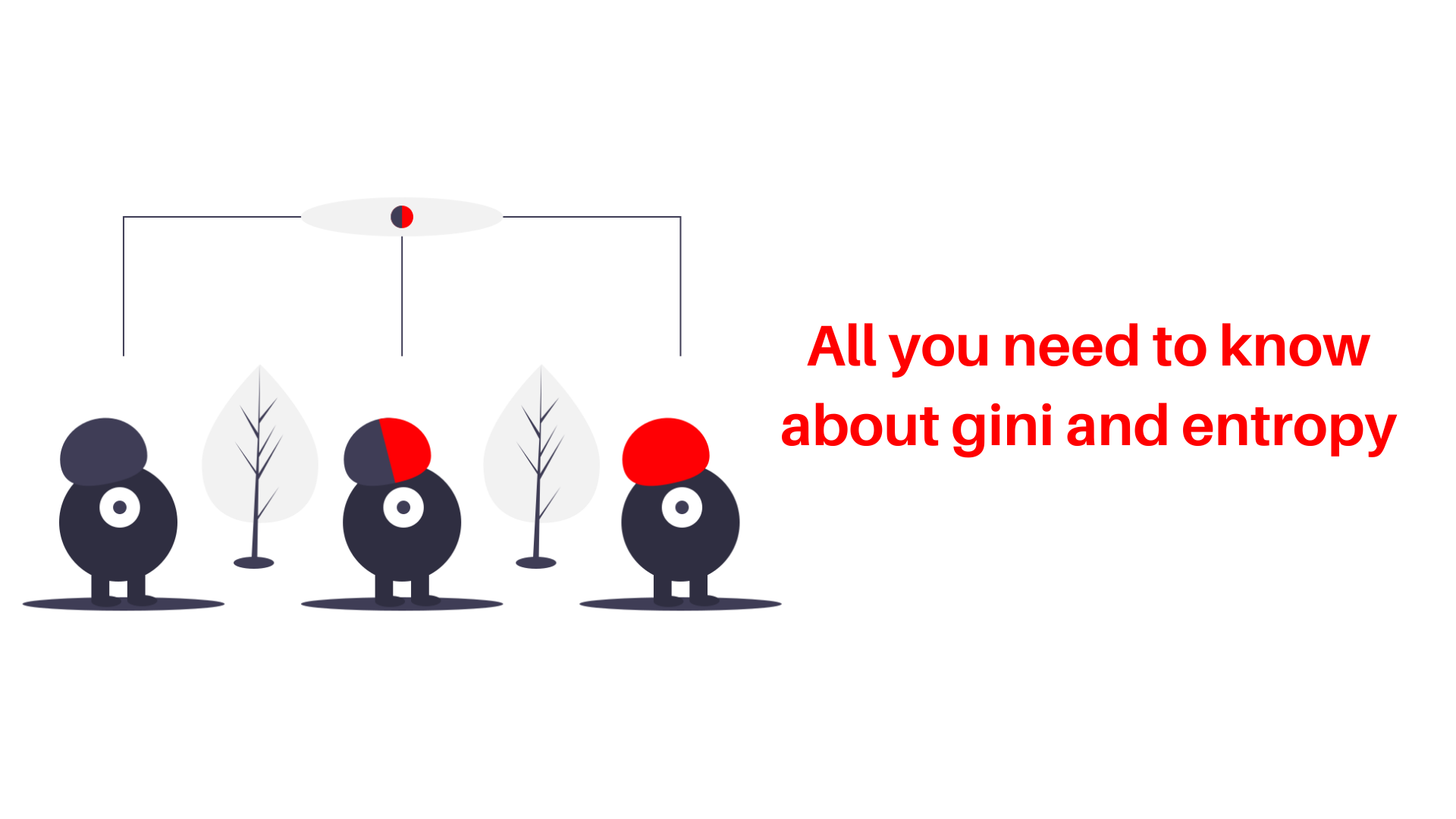# Decision Tree Gini vs entropy: Which one is better?Decision Tree Gini vs Entropy explains the impurity in your data split in a Decision Tree classifier. The algorithmic tree works just like a real tree with branches. Just like leaves grow on the branches, similarly, the leaf nodes grow out of the decision nodes on the branch of a Decision Tree. Every second section of a Decision Tree is therefore called a “branch.” An example of this is when the question is, “Are you a diabetic?” and the leaf nodes can be ‘yes’ or ‘no’.

## Decision Tree Explained

Decision Trees are powerful machine learning algorithms that can be used for both Regression and Classification problems. The essence of Decision trees is that a problem statement is taken by the algorithm and given classes of choices. The algorithm connects questions to choices and ends with a tree-like system where every branch is split into nodes and ultimately to leaf nodes where the decision is made. The decision of the split depends on the criterion being used which can be chosen from Gini and Entropy.

• A Root node is at the base of the decision tree.
• The process of dividing a node into sub-nodes is called Splitting.
• When a sub-node is further split into additional sub-nodes it is called a Decision node.
• When a sub-node depicts the possible outcomes and cannot be further split it is a Leaf node.
• The process by which sub-nodes of a decision tree are removed is called Pruning.
• The subsection of the decision tree consisting of multiple nodes is called Branches.

Also, read -> Decision Trees Loss Function

## Gini Impurity

Gini impurityGini’s diversity index or the Gini-Simpson index is generally used by classification and regression tree algorithms for Decision Trees, and therefore the Gini impurity (which is named after an Italian mathematician, Corrado Gini) is in simple terms, a measure of how frequently can some randomly chosen element from the set be incorrectly labeled if it is randomly labeled according to a distribution of labels.

Gini impurity can be computed by summing a squared given probability of an item ‘p’ with a label ‘i’ which is chosen ‘n’ times the probability minus ‘one’ giving the formula for Gini as given below of a mistake in categorizing that item. It reaches its minimum (zero) when all cases in the node fall into a single target category.

Gini impurity can have a value ranging from 0 to 0.5 which is the minimum and the maximum value respectively.

To use Gini in your code with Python’s Scikit Learn library, use the following code:

```from sklearn.tree import DecisionTreeClassifier
tree1 = DecisionTreeClassifier(random_state=0, criterion= 'gini')```

## Entropy

Entropy for any random variable or process is given as the average level of uncertainty involved in the possible outcome of the variable or process. Gini and Entropy are important to allow information gain. Let us take the probability of a coin flip where there are two probabilities of a head or a tail and if the probability of tail after flip is p then the probability of a head is given by 1-p. And the maximum uncertainty for p can be ½  when there is no reason to expect one outcome more than another. Hence, we can say that the entropy here is 1 and if an event is a known event and the maximum uncertainty is for p=0 or p=1 entropy is 0. The formula for entropy can be given by:

One can calculate the value of Information gained after calculating the Entropy. The formula for information gain is simple:

Information Gain = 1 – Entropy

Use the following code to create a decision tree instance with Entropy as the impurity measure:

```from sklearn.tree import DecisionTreeClassifier
tree1 = DecisionTreeClassifier(random_state=0, criterion= 'entropy')```

Entropy is a good measure of impurity alternating to the Gini Index as it considers the uncertainty involved in a choice.

## Conclusion

Both Gini Index and Entropy are highly preferred criteria when choosing the method to split the branches in a Decision tree. Gini and entropy, as already mentioned, are measures of impurity of a node. A node with multiple classes is impure and a node having only one class is considered pure. In general, there is hardly any difference between the performance of the Gini Index and Entropy on the same data and therefore the decision of what to use is left to the data scientist. The decision tree can make perfect predictions but the only catch is that it may then be overfitting on the data and can result in a huge tree with too many branches which won’t perform as expected on a test data or a new dataset that it has not worked on before and therefore the number of splits is also important to be considered when deciding which impurity measure to take.

Try using both Gini and Entropy and check which one offers the better performance for your tree!

For more such content, check out our blog -> Buddy Programmer